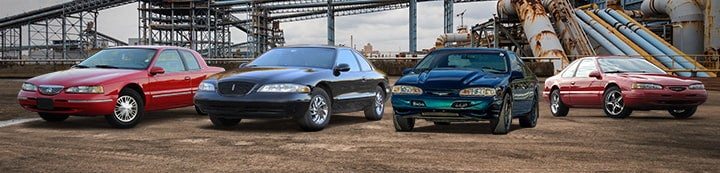1 - 4 of 4 Posts

#### paintme205

·
##### Registered
Joined
·
836 Posts
Discussion Starter
Since math isn't my strong point, I figured I would ask the experts.

I am trying to figure out how to calculate the difference in pulley rpms when you change the belt size on a thunderbird.

For example (all hypothetical)

7 inch main pulley
3 inch alternator
5 inch ac pulley
6 inch water pump pulley
84 inch belt
= what rpm for water pump and alternator

Now exchange that for a 65 inch belt and recalculate.

Any help or onllne calculators?

#### master486

·
##### Newbie
Joined
·
5,650 Posts
Just because you have a 4.6L doesn't mean every thread belongs there!

And about your question, belt length doesn't matter. What matters are the pulley diameters, or ratios. If the driving force is on the 7 inch pulley and the alternator pulley is 3 inches, there is a 7 ÷ 3 = 2.33 drive ratio, so take any RPM and multiply it by the drive ratio (i.e. 750 crank RPM * 2.33 = 1747.5 alternator RPM).

#### NetKeym

·
Joined
·
5,862 Posts
Since math isn't my strong point, I figured I would ask the experts.

I am trying to figure out how to calculate the difference in pulley rpms when you change the belt size on a thunderbird.

For example (all hypothetical)

7 inch main pulley
3 inch alternator
5 inch ac pulley
6 inch water pump pulley
84 inch belt
= what rpm for water pump and alternator

Now exchange that for a 65 inch belt and recalculate.

Any help or onllne calculators?
The length of the drive belt does NOT change the RPM of the pulleys at all (or the size of the pulleys, technically). The speed of the accessories is determined by the RATIO's of each pulley related to the only drive pulley (the crank or main pulley, as you put it).

So, lets use your examples with an idle of 650 RPM. The ratio of the alternator to main pulley is 3:7 or (7/3 = 2.33) 1:2.33. Therefore the rpm of the alternator is 650 x 2.33 = 1516.66 RPM. The water pump is 6:7 or 1:1.167 = 758.33 RPM.

I know it sounds like it shouldn't be that simple, but circumference = 2 x pi x radius. Well, 2 x radius = diameter and pi is a constant, so it IS that simple.

BTW, if you go with underdrive pulleys, the rule of thumb for the alternator pulley is 3:1. Thus, if you go to a 5" crank pulley (like I did), you should use a 5/3 = 1.67" alternator pulley (which ironically happens to be the diameter of the March 110 Alternator overdrive pulley they sell).

EDIT: Aw, Chris - you edited your post before I got mine out! lol

#### paintme205

·
##### Registered
Joined
·
836 Posts
Discussion Starter
Just because you have a 4.6L doesn't mean every thread belongs there!

And about your question, belt length doesn't matter. What matters are the pulley diameters, or ratios. If the driving force is on the 7 inch pulley and the alternator pulley is 3 inches, there is a 7 ÷ 3 = 2.33 drive ratio, so take any RPM and multiply it by the drive ratio (i.e. 750 crank RPM * 2.33 = 1747.5 alternator RPM).
Point noted.

Thanks for the help. I was trying to figure out if belt length mattered or not. I see now that it doesn't.

1 - 4 of 4 Posts